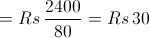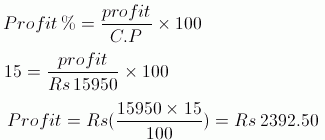## Wednesday, 25 December 2013

### CBSE Class 8 - Maths - Comparing Quantities (Ex 8.2)

Comparing Quantities

Q1:A man got a 10% increase in his salary.If his new salary is 1,54,000,find his original salary.

Let original salary be x. Given that new salary is 1,54,000.

Original salary + increment= New salary

But it is given that increment is 10% of the original salary.

Therefore,Thus,the original salary was Rs 1,40,000.

Q2: On Sunday 845 people went to the zoo.On Monday only 169 people went.What is the percent decrease in the people visiting zoo on Monday?

Given that on Sunday,845 people went to the zoo and on Monday,169 people went.

Decrease in number of people = 845-169=676

Percentage DecreaseQ3: A shopkeeper buys 80 articles for Rs 2,400 and sells them at the profit of 16%. Find the selling price of one article.

Since shopkeeper buys 80 articles for Rs 2.400,So

Cost of one articleProfit percent = 16Selling price of one article= C.P + Profit = Rs (30 + 4.80)= Rs. 34.80

Q4: The cost of an article was Rs 15,500. Rs 450 was spent on its repairs.If it is sold for a profit of 15%,find the selling price of the article.

Total cost of an article = Cost + Overhead expanses

= Rs 15500 + Rs 450

= Rs 15950∴ Selling price of one article= C.P + Profit = Rs(15950 + 2392.50)

=Rs 18342.50

Q5: A VCR and TV were bought for Rs 8,000 each.The shopkeeper made a loss of 4% on the VCR and profit of 8% on the TV.Find the gain or loss percent on the whole transaction.

C.P of a VCR = Rs 8,000

Shopkeeper made a loss of 4% on the VCR means if C.P = Rs 100 , then S.P is Rs 96

When C.P is Rs 8,000,S.PC.P of a TV= Rs 8000

Shopkeeper made a profit of 8% on the TV means if C.P = Rs 100 , then S.P is Rs 108

When C.P is Rs 8000,S.PTotal S.P = Rs 7680 + Rs 8640 = Rs 16320

Total C.P = Rs 8000 + Rs 8000 = Rs 16000

We can see that total S.P > total C.P ,this means there was a profit.

Total Profit = Rs 16320 - Rs. 16000 = Rs 320Therefore,shopkeeper had a gain of 2% on the whole transaction.

Q6: During a sale,a shop offered discount of 10% on the marked price of all the items.What would a customer have to pay for a pair of jeans marked at Rs 1450 and two shirts marked at Rs 850 each?

Total marked price = Rs (1450 + 2 x 850) = Rs (1450 + 1700) = Rs 3150

Given Discount %= 10 %Also, Discount = Marked Price - Sale Price

Rs 315= Rs 3150- Sale Price

∴ Sale Price = Rs (3150-315) = Rs 2385

Thus, customer have to pay Rs 2,385

Q7: A milkman sold two of his buffaloes for Rs 20,000 each.On one he made a gain of 5% and on the other a loss of 10%.Find his overall gain or loss.

S.P of each buffalo = Rs 20000

Milkman made a profit of 5% on selling one buffalo means if C.P = Rs 100 , then S.P is Rs 105

C.P of one buffaloAlso,Milkman made a loss of 10% on selling second buffalo means if C.P = Rs 100 , then S.P is Rs 90

∴ C.P of other buffaloTotal C.P = Rs 19047.62 + Rs 22222.22 = Rs 41269.84

Total S.P = Rs 20000 + Rs 20000 = Rs 40000

We can see that total C.P > total S.P ,this means there was a loss.

Loss = Rs 41269.84 - Rs 40000 = Rs 1269.84

So,overall loss of milkman was Rs 1269.84

Q8: The price of a TV is Rs 13,000.The sales tax charged on it is at the rate of 12%. Find the amount that Vinod will have to pay if he buys it.

On Rs 100,the tax to be paid = Rs 12

On Rs 13000,the tax to be paid will be = Rs. 12/100 × 13000
= Rs 1560

Required amount = Cost + Sales Tax = Rs 13000 + Rs 1560

= Rs 14560

So,Vinod have to pay Rs 14560 for the TV.

Q9: Arun bought a pair of skates at a sale where the discount given was 20%. If the amount he pays is Rs 1,600 , find the marked price.

Let the marked price be x.Also,

Discount = Marked Price - Sale PriceSo,the marked price was Rs 2000

Q10: I purchased a hair-dryer for Rs 5,400 including 8% VAT. Find the price before VAT was added.

The price includes VAT which means that if the price without VAT is Rs 100, then price including
VAT will be Rs 108.

When price including VAT is Rs 108 ,Original price = Rs 100

When price including VAT is Rs 5400 ,Original priceSo,the price of hair-dryer before the addition of VAT was Rs 5,000.1.2.